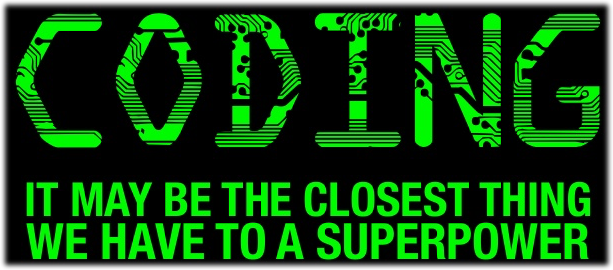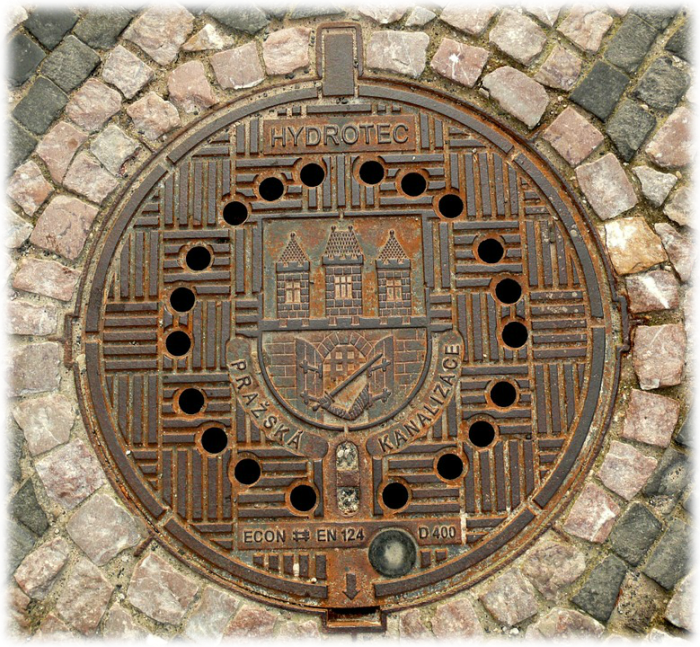C# Elementary6 Talk in the big kitchenIntroduction to double type# Behavior as Usual

My mood was much better after visiting the basement. In this dark and grim world, I finally had at least one hope to hold onto - the hope that there was at least a chance that I could make it back to my own time.
For a few days after getting into the basement and back out again, I engaged in some people watching whenever I was moving around the base. By the looks of it, no one had particularly noticed my absence, which meant that I should be able to continue helping Noname to restore his modules without drawing suspicion.

Jessica was heading toward me in the corridor. "Hi, Teo. You look so enthusiastic today!"
"Thanks, you look pretty chipper yourself!" I returned. I suddenly noticed that everyone other than me was headed in the other direction. "Where's everyone going?" I asked.
"Ritchie returned from an expedition and said he had something to show off. He called a meeting in the big kitchen at..." Jessica looked at her watch, "Now! Let's go!" I turned on my heels and tried to keep up.

# The Types

Ritchie was standing at the front of the kitchen, and most of the tables were full. People were still trickling in little by little, and everyone was waiting for him to begin. Finally:
"Hello, everyone! Thank you all for coming on such short notice. I've just returned from the machine city, and an ally of ours shared some intel they've been working on obtaining for a while. It turns out there are many more types than just bool , int , and string .

"More? Really?" Someone from the crowd called. Ritchie replied, "Yes, many more. There are so-called built-in types , which are more complex types than string and the other types we already know. Let's start simple and go over some of the built-in types, the first of which are the float and double types. Both of these types represent a real number, sometimes referred to as a floating-point number (this is where the name 'float' goes from). For example," at this, Ritchie uncapped a marker and began writing on the whiteboard behind him, "numbers like 2.5, 17.9456, and 0.7 are real numbers."

"Why would we need such complexity?" someone asked, mirroring my own thoughts. "I use int in all my programs and everything seems to work fine!"

Ritchie had clearly anticipated this question. "In our world, the primary currency is viruses. Every number of viruses is an integer number. You can have 2, 5, or 10 viruses, but not 2.5, right? That's why you think in terms of the int type. Before our time though, people had a currency - the dollar - to calculate their money. You could have 1.50 dollars, or 1.63 dollars, not just 1 or 2. In a similar manner, you can use a double variable to store a real number inside:"

``````double myMoney = 187.543;
``````

He continued, "I know it seems complicated, but you can add, subtract, divide, and multiply double variables same as you do with integers."

``````double myMoney = 10.25;

double fourTimesMyMoney = 10.25 * 4.0;
Console.WriteLine(fourTimesMyMoney);        // Outputs 41

double decreasedMoney = myMoney - 1.25;
Console.WriteLine(decreasedMoney);          // Outputs 9

double dividedMoney = decreasedMymoney / 2;
Console.WriteLine(dividedMoney);            // Outputs 4.5

double addToMyMoney = dividedMoney + 100.34;
``````

Ritchie continued making the case for the new types. "Even better," he said, "you can add, subtract, divide, and multiply double and int variables together. The result is automatically converted to a double type."

``````double someDouble = 7.5;
int someInt = 2;

double sum = someDouble + someInt;
Console.WriteLine(sum);                 // Outputs 9.5

double subtract = someDouble - someInt;
Console.WriteLine(subtract);            // Outputs 5.5

double multiply = someDouble * someInt;
Console.WriteLine(multiply);            // Outputs 15

double divide = someDouble / someInt;
Console.WriteLine(divide);              // Outputs 3.75

``````

This isn't any different than how you do things outside of coding. We can add integers and real numbers, subtract them, divide them, and multiply them without any hiccups. At the same time, you cannot multiply strings with any other type, or divide or subtract. You can only add strings (using "+") to numeric types . Here are some examples:

``````double someDouble = 7.5;
int someInt = 2;
string someString = "Hello ";
double result1 = someDouble + someInt; // Ok - adding double and int
string result2 = someString + someDouble; // Ok - append double to string
string result3 = someString + someInt; // Ok - append int to string
string result4 = someString * someInt; // NOT ok - can't multiply int and
// string
string result5 = someString / someDouble; // NOT ok - can't divide string on
// double
string result6 = someString * someString; // NOT ok - can't multiply strings
``````

To output a double to the screen or concatenate it with a string, you use exactly the same mechanisms as you used with an int .

``````double temperature = 17.5;
Console.WriteLine(temperature); // Outputs: 17.5
Console.WriteLine("Temp is " + temperature); // Outputs: Temp is 17.5
Console.WriteLine(\$"Temp is {temperature}"); // Outputs: Temp is 17.5
``````

Everyone in the room was nodding. Ritchie asked us to try creating a double variable and then output it to the screen.

If you want to read a double from the screen, use the method double .Parse(...) the same as you used int .Parse(...). Here is an example:

``````string numberString = Console.ReadLine();
double number = double.Parse(numberString);
``````A float is almost the same as a double . The main difference is that a double is twice as large as a float in memory space (hence the name 'double'). This results in a different range of values that you can store in these two types:

Type Space in Memory Approximate Range Precision Default Value Example
float 4 bytes -3.4 x 10 38 to +3.4 x 10 38 7 0.0 f 0.1234567 f
double 8 bytes ±5.0 x 10 -324 to ±1.7 x 10 308 16 0.0 0.1234567890123456

By default, every real number in C# program is treated as a double . To force C# to recognize a number as a float, you must add an "f" at the end of every float, as shown in the above table and the below example.

You can add, subtract, multiply, and divide double and float variables the same as you can with doubles and ints. The result will again be a double type.

``````double someDouble = 1.5;
float someFloat = 2.5f;

double sum = someDouble + someFloat;
Console.WriteLine(sum);                 // Outputs 4

double subtract = someDouble - someFloat;
Console.WriteLine(subtract);            // Outputs -1

double multiply = someDouble * someFloat;
Console.WriteLine(multiply);            // Outputs 3.75

double divide = someDouble / someFloat;
Console.WriteLine(divide);              // Outputs 0.6
``````

After walking around to make sure we had grasped the new concepts, Ritchie returned to the front of the room. "From now on," he began, "we're going to use the double type because it's as fast as the float type, but provides much more precise results. I suggest that you all get comfortable using the double in your programs, and only use a float if you have a very good reason to do so."

"Let's wrap up the first part of our meeting," Ritche said. "Complete this next task and then take a half-hour lunch break. We'll get into more new types this afternoon!"At Codeasy we believe that learning should be fun and engaging. Please reach out to us to share your feedback and collaboration ideas.